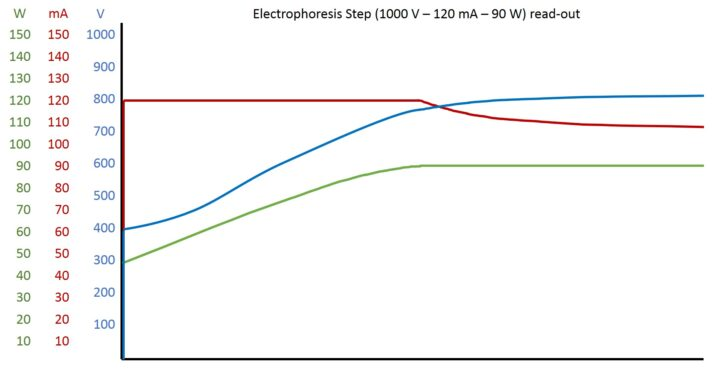Parameter during electrophoresis – the cross-over

Why are the programmed parameters not reached during electrophoresis?

This is due to the cross-over effect, which we want to explain in this post.An electrophoresis read-out of our BluePower power

supply should serve as an example. An electrophoresis run with three 2D HPE™ Large gels on an HPE™ BlueHorizon™ Triple Deck was recorded.

The programmed parameters were 1000 V, 120 mA, 90 W (See graph on the right).

The programmed parameters are not the supplied values. Rather, these are limitations and therefore only maximum permitted values. Using the physical formula to calculate the power, a first calculation shows that with the programmed voltage and current values, the power of 90W is exceeded:

P = U x I = 1000 V x 0,120 A = 120 W

Why choosing a lower power value?

The watts equate to the Joule heat dissipation. The power value is chosen to ensure that the active or passive cooling can compensate this heat dissipation. If the gel gets too warm, it dries out and can simmer (flat bed gels) or the matrix melts (vertical gels). Both lead to poor separations.

Theoretically, one can term three prominent points during an electrophoresis run: start, cross-over, end. It is called cross-over because the parameters replace one another as constants.

Electrophoresis start:

Current increases proportionally to the ion flow. The ion flow is high when many ions are present. Since all the ions are present in the buffer at the beginning of the electrophoresis, the ion flow and thus the current value are at a maximum. Since the limitation is programmed to 120 mA, the current immediately increases to this value. Volts and watts adapt to this value consistently. In our example, voltage starts at approx. 380 V. The product of current and voltage then results in a power value of 45 W.

Cross-over:

The ion flow decreases steadily as more and more ions of the buffer reach the electrodes and are no longer available to the system. This is not yet observable from the current values, because the limitation is still smaller than the theoretically possible current value. However, one can notice that the steadily decrease of ion flow makes higher voltages, and thus also watts, possible. Volt and watt values increase steadily until power has reached its limit (90 W). With ongoing electrophoresis, the current value will drop below the limit and steadily decrease. Voltage values continue to rise. The above formula can be used to calculate the current value at which the voltage limit is reached at constant watts.

I = P / U = 90 W / 1000 V = 90 mA

Electrophoresis end:

If enough ions remain, the voltage limit is reached and the current and power values decrease over time. Depending on the electrophoresis application and system, it is possible that the current value is decreasing too slow and the voltage limit is not reached in given time. Since the seperation distance of the proteins is proportional to voltage, it is usually necessary that a high voltage value is reached for a certain period of time. To overcome slow voltage increase, one possibility is to program a following step in which parameters are raised.